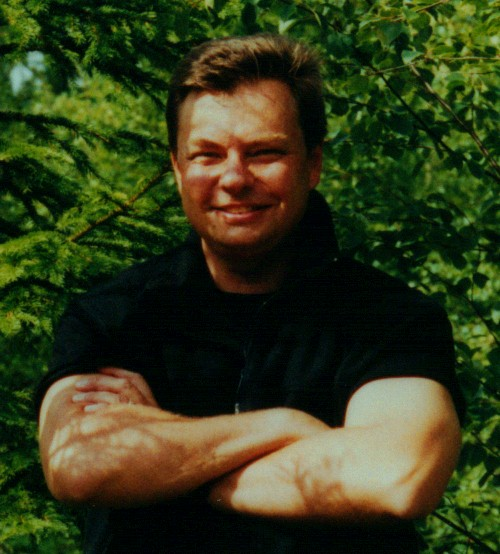http://www.sekon.SLU.se/~PLOGeneral historical background:
The name "FAUSIMP1" is constructed from "Faustmann", "Simple" and "Version 1". Martin Faustmann wrote his famous forest economics article in 1849. He did not consider risk, uncertainty, constraints or economies of scale. Still, the model by Faustmann has been very dominating during 150 years. In the same way, the mathematical principles discovered by Newton have been very dominating for hundreds of years. It is well known that Einstein and Heisenberg made new fundamental discoveries long ago. Still the Newton ideas can be used with good results in many applications of today. If you consider risk, uncertainty, constraints or economies of scale, you may find even better solutions in some cases. Please visit my web page http://www.sekon.SLU.se/~PLO  for more information and software!

General problem description:
Right now, you have a forest stand. The age of the stand is approaching the age of the final felling. The only remaining forest management activity in the stand is the final felling. Now, you can optimize the economic result of your harvest decision in a very simple way. Here, it is assumed that everything is known with certainty. (This can of course be questioned! Compare the other models and information found from  http://www.sekon.SLU.se/~PLO.) This model is based on classical maximization of the present value via selection of the harvest year. It is assumed that the following information is known:
The volume per hectare right now, v0, the growth of the volume per hectare and year, v1, the net price (= price - harvest costs) per cubic metre right now, p0, the growth of the net price per cubic metre per year, p1, the value per hectare of the bare land released after harvest, L, and the rate of interest (%) in the capital market, r.

1. How many years from now should I harvest my forest stand in order to maximize the present value per hectare?
2. How high is the maximum present value per hectare?
3. Which is the present value per hectare if I harvest at some other point in time, "tev" years from now?

In the java program below, you enter information in a table. The information is v0, v1, p0, p1, L, r and tev.
The volume per hectare, V(t), is a function of time, t. V(t) = v0 + v1*t  (t = 0 right now and increases one step each year)
The net price per cubic metre, P(t), is a function of time, t. P(t) = p0 + p1*t
L is the value per hectare of the bare land released after harvest.
The rate of interest in continuous time, r, is defined in the unit %.
An arbitrary harvest year, which you suggest, is denoted by tev.

When the table is full, just press the "SOLVE" button and your results appear below the table!
If you want to modify some data in the table, just do that and press "SOLVE" again!
Note: If the "Optimal harvest year" turns out to be negative, such as -5, this means that you should have cut 5 years ago!

Example solution:
Assume that you enter the following data: v0 = 160, v1 = 5, p0 = 190, p1 = 1, L = 2000, r = 3, tev = 40.
(We may use the currency in your country, such as \$US, SEK, £, or anything else. You should not enter the currency in the table. In this section, we just assume that the currency is SEK.)
Then you should get the following results:
The optimal harvest year occurs about 6 years from now.
The optimal (maximum) present value is 32777 SEK/hectare.
Your suggested harvest year, tev, 40 years from now, would give the present value 25541 SEK/hectare. That is much worse than the maximum value 32777 SEK/hectare.

Welcome to my home page http://www.sekon.SLU.se/~PLO for more information and software! There you can find much more about optimal decisions under risk, uncertainty, constraints, economies of scale, continuous cover forestry, plantations and more!

Peter Lohmander